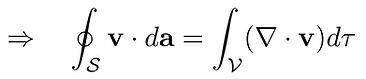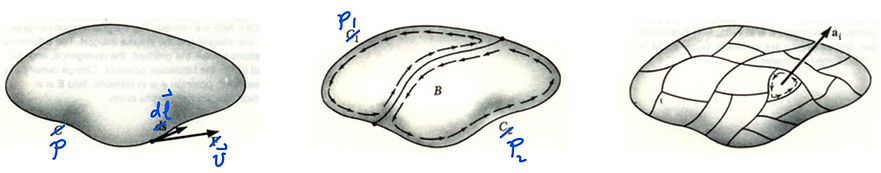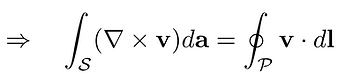top of page

## The fundamental theorem for divergences (Divergence theorem, Gauss's theorem)Recalling the definition of divergence,## The fundamental theorem for curls (Stokes' theorem)Recalling the definition of curl,bottom of page